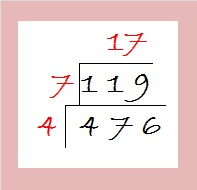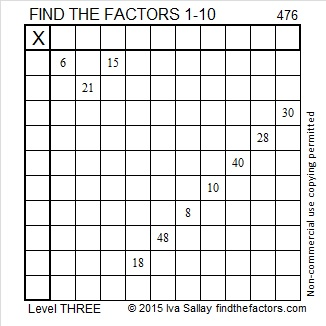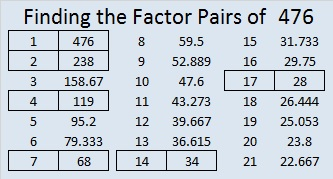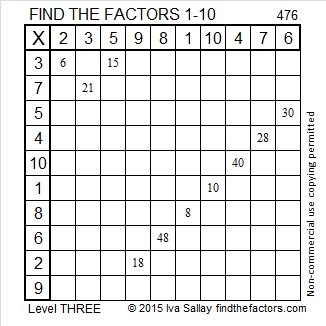# 476 and Level 3

76 can be evenly divided by 4 so 476 is also divisible by 4. Since 476 has a square factor, √476 can be simplified. Reducing square roots is easy if you make a cake: First divide 476 by its smallest square factor, 4, to get 119, a number that doesn’t have any square factors. Dividing 119 by its smallest prime factor is optional, but can help you see that 119, indeed, has no square factors. Last of all, take the square root of everything on the outside of the cake and multiply them together. √476 = (√4)(√(7 x 17) = (√4)(√119) = 2√119.Here is today’s factoring puzzle:Print the puzzles or type the solution on this excel file: 10 Factors 2015-04-27

—————————————————————————————————

• 476 is a composite number.
• Prime factorization: 476 = 2 x 2 x 7 x 17, which can be written 476 = (2^2) x 7 x 17
• The exponents in the prime factorization are 2, 1, and 1. Adding one to each and multiplying we get (2 + 1)(1 + 1)(1 + 1) = 3 x 2 x 2 = 12. Therefore 476 has exactly 12 factors.
• Factors of 476: 1, 2, 4, 7, 14, 17, 28, 34, 68, 119, 238, 476
• Factor pairs: 476 = 1 x 476, 2 x 238, 4 x 119, 7 x 68, 14 x 34, or 17 x 28
• Taking the factor pair with the largest square number factor, we get √476 = (√4)(√119) = 2√119 ≈ 21.81742—————————————————————————————————

A Logical Approach to solve a FIND THE FACTORS puzzle: Find the column or row with two clues and find their common factor. Write the corresponding factors in the factor column (1st column) and factor row (top row).  Because this is a level three puzzle, you have now written a factor at the top of the factor column. Continue to work from the top of the factor column to the bottom, finding factors and filling in the factor column and the factor row one cell at a time as you go.This site uses Akismet to reduce spam. Learn how your comment data is processed.